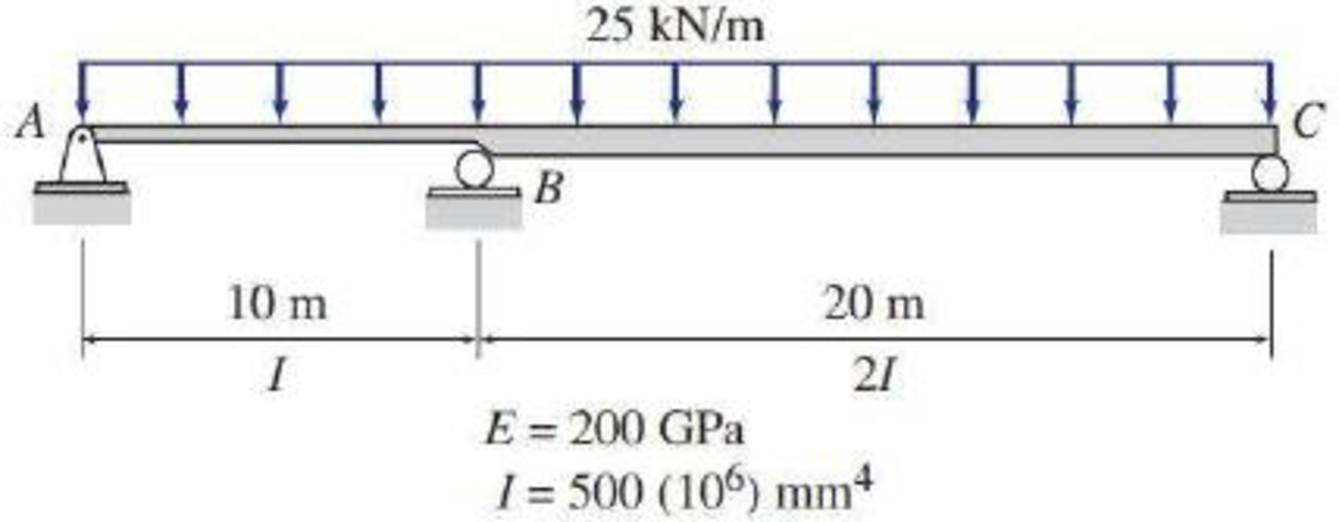# Solve Problem 13.12 for the loading shown in Fig. P13.12 and the support settlements of 10 mm at A , 65 mm at B , and 40 mm at C . 13.9 through 13.12 Determine the reactions and draw the shear and bending moment diagrams for the beams shown in Figs. P13.9–P13.12 using the method of consistent deformations. Select the reaction at the interior support to be the redundant.

#### Solutions

Chapter
Section
Chapter 13, Problem 51P
Textbook Problem
22 views

## Solve Problem 13.12 for the loading shown in Fig. P13.12 and the support settlements of 10 mm at A, 65 mm at B, and 40 mm at C.13.9 through 13.12 Determine the reactions and draw the shear and bending moment diagrams for the beams shown in Figs. P13.9–P13.12 using the method of consistent deformations. Select the reaction at the interior support to be the redundant.To determine

Find the reactions and sketch the shear and bending moment diagrams for the given beam using method of consistent deformation.

### Explanation of Solution

Given information:

The settlement at support A (ΔA) is 10 mm.

The settlement at support B (ΔB) is 65 mm.

The settlement at support C (ΔC) is 40 mm.

Apply the sign conventions for calculating reactions, forces and moments using the three equations of equilibrium as shown below.

• For summation of forces along x-direction is equal to zero (Fx=0), consider the forces acting towards right side as positive (+) and the forces acting towards left side as negative ().
• For summation of forces along y-direction is equal to zero (Fy=0), consider the upward force as positive (+) and the downward force as negative ().
• For summation of moment about a point is equal to zero (Matapoint=0), consider the clockwise moment as negative and the counter clockwise moment as positive.

Calculation:

Show the beam as shown in the Figure 1.

Refer Figure 1.

Consider the horizontal and vertical reaction at A are denoted by Ax and Ay.

Consider the vertical reaction at B and C is denoted by By and Cy.

The reactions acting in the beam is 4.

The number of Equilibrium reaction is 3.

The degree of indeterminacy of the beam is 1.

Take the vertical reaction at B as the redundant.

Modify the Figure 1 as shown in Figure 2.

Refer Figure 2.

Consider a section at a distance x from both the supports A and C.

The deflection at B due to the external loading is denoted by ΔBO.

The flexibility coefficient representing the deflection at B due to unit value of redundant By is fBB.

Sketch the settlement at supports as shown in Figure 3.

Refer to Figure 3.

The settlement at B ΔB=6520=45mm

Primary beam subjected to unit value of redundantBy.

Calculate the value of reaction at C as follows:

MA=0Cy×30+1×10=0Cy=13

Calculate the value of reaction at A as follows:

V=0Ay+Cy=1Ay+13=1Ay=23

Calculate the value of deflection fBB as follows:

fBB=1EI010(23x)2dx+12020(13x)2dx=1EI01049x2dx+1202019x2dx=1EI[(49×x33)010+(12×19×x33)020]=1EI[(49×1033)+(12×19×2033)]

=1EI[148.1481+148.1481]=296.3kNm3/kNEI        (1)

Substitute 200GPa for E and 500×106mm4 for I in Equation (1).

fBB=296.3kNm3/kN200GPa×106kN/m21GPa×500×106mm4×(1m1,000mm)4=296.3100,000=0.00296m/kN×1,000mm1m=2.96mm/kN

Calculate the value of reaction at A and C as follows:

Ay=Cy=25×302Ay=Cy=375kN

Calculate the value of deflection ΔBO as follows:

ΔBO=1EI[010(375x25x22)(23x)dx+12020(375x25x22)(13x)dx]=1EI[010(250x2+50x36)dx+12020(125x2+25x36)dx]=1EI[(250×x33+50x424)010+12(125x33+25x424)020]=1EI[(250×1033+50×10424)+12(125×2033+25×20424)]

=1EI[(250×1033+50×10424)+12(125×2033+25×20424)]=1EI[62,50083,333

### Still sussing out bartleby?

Check out a sample textbook solution.

See a sample solution

#### The Solution to Your Study Problems

Bartleby provides explanations to thousands of textbook problems written by our experts, many with advanced degrees!

Get Started

Find more solutions based on key concepts
Obtain the EPAs consumer fact sheets (they are now available on the Web) on antimony, barium, beryllium, cadmiu...

Engineering Fundamentals: An Introduction to Engineering (MindTap Course List)

What is a single sign-on account?

Enhanced Discovering Computers 2017 (Shelly Cashman Series) (MindTap Course List)

Describe the DBAs responsibilities.

Database Systems: Design, Implementation, & Management

What is a managerial control?

Management Of Information Security

What are the two basic types of tapping toolholders?

Precision Machining Technology (MindTap Course List)

The magnitude of the moment of the force P about the axis CD is 50lbin. Find the magnitude of P.

International Edition---engineering Mechanics: Statics, 4th Edition

If your motherboard supports ECC DDR3 memory, can you substitute non-ECC DDR3 memory?

A+ Guide to Hardware (Standalone Book) (MindTap Course List)